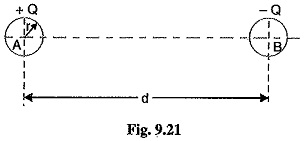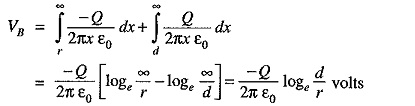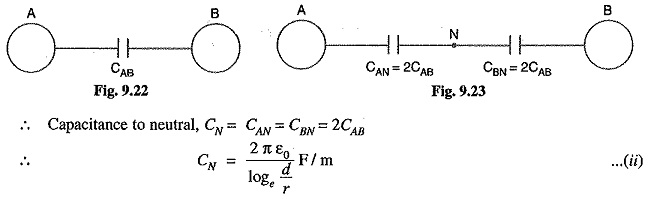## Capacitance of a Single Phase Two Wire Line:

Consider a Capacitance of a Single Phase Two Wire Line consisting of two parallel conductors A and B spaced d metres apart in air. Suppose that radius of each conductor is r metres is shown in Fig. 9.21. Let their respective charge be + Q and – Q coulombs per metre length.The total p.d. between conductor A and neutral “in­finite” plane isSimilarly, p.d. between conductor B and neutral “infinite” plane isBoth these potentials are w. r. t. the same neutral plane. Since he unlike charges attract each other, the potential difference between the conductors is### Capacitance to Neutral:

Equation (i) gives the capacitance between the conductors of a two-wire line [See Fig. 9.22]. Often it is desired to know the capacitance between one of the conductors and a neutral point between them. Since potential of the mid-point between the conductors is zero, the potential difference between each conductor and the ground or neutral is half the potential difference between the conductors. Thus the capacitance to ground or capacitance to neutral for the two-wire line is twice the line-to-line capacitance (capacitance between conductors as shown in Fig 9.23).The reader may compare eq. (ii) to the one for inductance. One difference between the equations for capacitance and inductance should be noted carefully. The radius in the equation for capacitance is the actual outside radius of the conductor and not the GMR of the conductor as in the inductance formula. Note that eq. (ii) applies only to a solid round conductor.

Scroll to Top